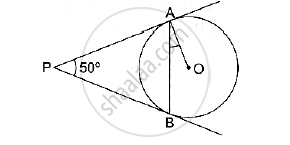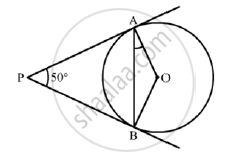# In the Given Figure, Pa and Pb Are Two Tangents to the Circle with Centre O. If ∠Apb = 50° Then What is the Measure of ∠Oab. - Mathematics

In the given figure, PA and PB are two tangents to the circle with centre O. If ∠APB = 50° then what is the measure of ∠OAB.#### Solution

Construction: Join OBWe know that the radius and tangent are perpendicular at their point of contact
∴ ∠OBP = ∠OAP  = 90°
∠AOB + ∠OBP  + ∠APB + ∠OAP = 360°     [Angle sum property of a quadrilateral]
⇒ ∠AOB  + 90° + 50° + 90° = 360°
⇒ 230° + ∠BOC= 360°

⇒ ∠AOB = 130°
Now, In isosceles triangle AOB
∠AOB  + ∠OAB  +∠OBA =180°       [Angle sum property of a triangle]
⇒ 130° + 2∠OAB = 180°          [∵ ∠OAB =  ∠OAB]
⇒ ∠OAB = 25°

Concept: Concept of Circle - Centre, Radius, Diameter, Arc, Sector, Chord, Segment, Semicircle, Circumference, Interior and Exterior, Concentric Circles
Is there an error in this question or solution?